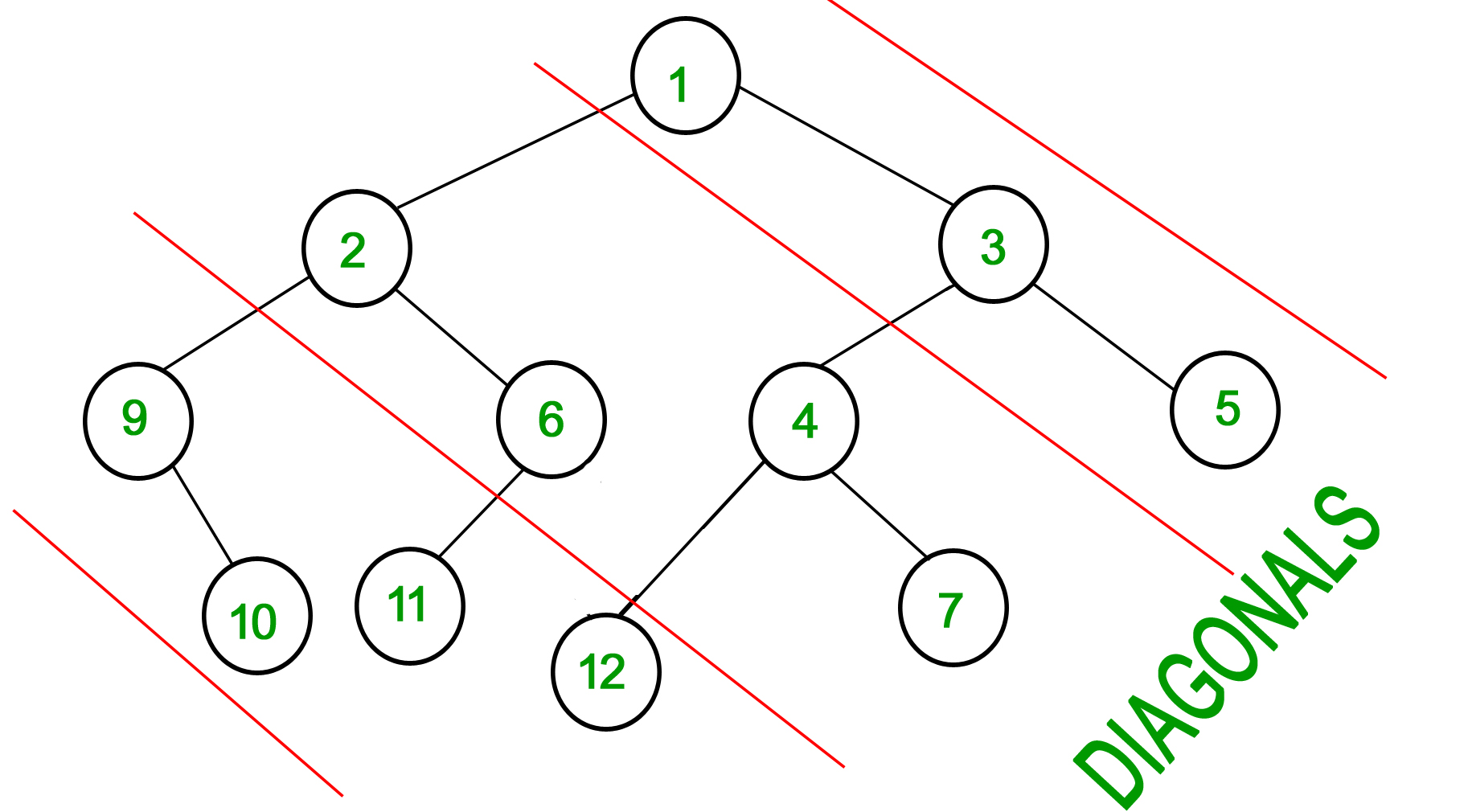Showing:HandleScore
@Ibrahim Nash 5725
@akhayrutdinov 5111
@mb1973 4989
@Quandray 4908
@saiujwal13083 4156
@sanjay05 3762
@marius_valentin_dragoi 3511
@sushant_a 3453
@KshamaGupta 3318
@the_coder95 3302
Diagonal Sum In Binary Tree
Easy Accuracy: 51.18% Submissions: 12283 Points: 2

Consider Red lines of slope -1 passing between nodes (in following diagram). The diagonal sum in a binary tree is the sum of all node’s data lying between these lines. Given a Binary Tree of size N, print all diagonal sums.

For the following input tree, output should be 9, 19, 42.
9 is sum of 1, 3 and 5.
19 is sum of 2, 6, 4 and 7.
42 is sum of 9, 10, 11 and 12.Example 1:

Input:
4
/   \
1     3
/
3
Output: 7 4


Example 2:

Input:
10
/    \
8      2
/ \    /
3   5  2
Output: 12 15 3


You don't need to take input. Just complete the function diagonalSum() that takes root node of the tree as parameter and returns an array containing the diagonal sums for every diagonal present in the tree with slope -1.

Expected Time Complexity: O(N).
Expected Auxiliary Space: O(N).

Constraints:
1<=Number of nodes<=105

Note: The Input/Ouput format and Example given are used for system's internal purpose, and should be used by a user for Expected Output only. As it is a function problem, hence a user should not read any input from stdin/console. The task is to complete the function specified, and not to write the full code.

### Editorial

We strongly recommend solving this problem on your own before viewing its editorial. Do you still want to view the editorial?

#### My Submissions: